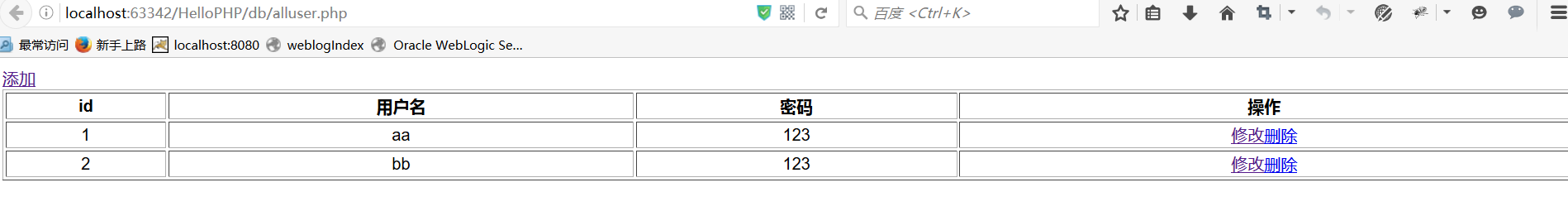# php简介

PHP是能让你生成动态网页的工具之一。PHP网页文件被当作一般HTML网页文件来处理并且在编辑时你可以用编辑HTML的常规方法编写PHP。
PHP代表：超文本预处理器（PHP: Hypertext Preprocessor）。PHP是完全免费的，不用花钱，你可以从PHP官方站点(http://www.php.net)自由下载。PHP遵守GNU公共许可（GPL)，在这一许可下诞生了许多流行的软件诸如Linux和Emacs。你可以不受限制的获得源码，甚至可以从中加进你自己需要的特色。PHP在大多数Unix平台，GUN/Linux和微软Windows平台上均可以运行。

PHP5.0版本强化更多的功能。首先，完全实现面向对象，提供名为PHP兼容模式的功能。其次是XML功能，PHP5.0版本支持可直观地访问XML数据、名为SimpleXML的XML处理用界面。同时还强化了XMLWeb服务支持，而且标准支持SOAP扩展模块。数据库方面，PHP新版本提供旨在访问MySQL的新界面——MySQL。除此前的界面外，还可以使用面向对象界面和预处理语句（Prepared Statement）等MySQL的新功能．另外，PHP5.0上还捆绑有小容量RDBMS-SQLite．

## 环境搭建

• cygwin:在windows中模拟linux的环境。
• apache httped：服务器。
• Nginx：服务器。
• xampp：应用服务器，快速搭建开发环境。
• phpStorm：php集成开发环境。
• ftp:协议，上传文件。
• ssh：一个命令，连接服务器。
• scp:一个命令，上传和下载文件。

# php基本语法

        <?php
echo 'hello php'

## 常量和变量、数组、函数

  $a=10;$a=20;
$b=5; echo$a+$b; 如果要定义常量的话可以使用：  const THE_VALUE=100; echo THE_VALUE; 也可以用函数来传值：  function traceNum($a,$b) { echo "a=$a,b=$b"; } traceNum(3,4); 常量只能被赋值一次，而变量可以赋值多次。 ## 流程控制、循环 php的流程控制可以使用if else来处理以及switch，和java类似，可以使用break和continue来控制循环. 下面是一个if循环的例子，这里注意的是elseif是连在一起写的，不要分开，和oracle中的存储函数类型，存储函数就是elsif来控制的，真的很像，呵呵！  function getLevel($score){
if($score>90){ return '优秀'; }elseif($score>80){
return '良好';
}elseif($score>60){ return '合格'; }else{ return '不合格'; } } echo getLevel(93); 如果使用switch的话可以这样：  function getLevel($score){

$result='不合格'; switch(intval($score/10)){
case 10;
case 9:
$result='优秀'; break; case 8:$result='良好';
break;
case 7:
$result='好'; break; case 6:$result='合格';
break;
default:
$result='不合格'; break; } return$result;

}
echo getLevel(93);

## 类、方法

 <?php

class Hello{
public function sayHello(){
echo 'hello';
}
}

    include  'demo1.php';  //包含，如果没有不会报错。
require  'demo1.php';  //依赖 ，如果没有就报错

//同一个php在不同的地方重复引用，
require_once  'demo1.php';

    class Man
{
/**
* @param $age年龄 * @param$name   名字
*
*/
public  function __construct($age,$name){
// echo 'Construce  a man';
$this->_age=$age;
$this->_name=$name;
}

public function getAge(){
return $this->_age; } public function getName(){ return$this->_name();
}
private $_age,$_name;

public static function sayHello(){
echo 'hello man';
}

}

## 库函数

 //获取时间
//echo  time();

date_default_timezone_set('Asia/Shanghai');
//日期
echo  date('Y-m-d H:i:s');


 //生成json格式的数据
$arr=array(1,2,3,5,7,'hello'); echo json_encode($arr);

$obj=array('h'=>'hello','w'=>'world',array(3,4,5,7)); echo json_encode($obj);

//解码
$jsonStr="{\"h\":\"hello\",\"w\":\"world\",\"0\":[3,4,5,7]}";$obj=json_decode($jsonStr); echo$obj->h;

    $img=imagecreate(400,300); imagecolorallocate($img,255,255,255);
header('Content-type:image/png');
imageellipse($img,200,200,50,50,imagecolorallocate($img,255,0,0));
imagepng($img); 为图片添加水印 $img=imagecreatefrompng('img.png');

imagestring($img,5,5,5,'www.tianfang1314.cn',imagecolorallocate($img,255,0,0));

header('Content-type:image/png');
imagepng($img); 操作文本 把数据写到data的文本中： $f=@fopen('data','w');
fwrite($f,'hello php'); fclose($f);

 $f=@fopen('data','r'); while(!feof($f)){
$content=fgets($f);
echo $content; } fclose($f);

 echo file_get_contents('data');

html页面端：

     <form  action="upload.php"  method="post"  enctype="multipart/form-data">
<input type="file"  name="file"  >
<input type="submit" value="提交" />
</form>

php端：

    $file=$_FILES['file'];
$fileName=$file['name'];
move_uploaded_file($file['tmp_name'],$fileName);

## 会话管理

 setcookie('name','tf');

    echo  $_COOKIE['name']; 如果用户禁用了cookie的话我们可以通过url来传值处理。 session: session_start();$_SESSION['name']='tf';

  session_destroy();

# mysql连接

 define('MYSQL_HOST','localhost:3306');   //mysql地址和端口号
define('MYSQL_USER','zp');   //用户名
define('MYSQL_PW','a');   //密码

function connecDB(){
$conn=mysql_connect(MYSQL_HOST,MYSQL_USER,MYSQL_PW); if(!$conn){
die('can\'t  connection db');
}

mysql_select_db('day15');   //数据库的名字
return  conn; } 使用php和html来生成一个表格。  <?php require_once 'config.php'; ?> <!DOCTYPE html> <html lang="en"> <head> <meta charset="UTF-8"> <title>Title</title> </head> <body> <a href="adduser.html" >添加</a> <table width='100%' style='text-align: center;' border='1' > <tr><th>id</th><th>用户名</th><th>密码</th><th>操作</th></tr> <?php /** * Created by PhpStorm. * User: asus * Date: 2016/8/26 * Time: 14:28 */conn=connecDB();
/* mysql_select_db("day15");*/
$result=mysql_query("select * from phpuser",$conn);
$dataCount=mysql_num_rows($result);
//  echo $dataCount; for($i=0;$i<$dataCount;$i++){$result_arr=mysql_fetch_assoc($result); //print_r($result_arr);

$id=$result_arr['id'];
$name=$result_arr['uname'];
$pwd=$result_arr['pwd'];

echo "<tr><td>$id</td><td>$name</td><td>$pwd</td><td><a href='updateuser.php?id=$id'>修改</a><a href='deleteuser.php?id=$id'>删除</a></td></tr>"; } ?> </table> </body> </html> 添加数据： $name=$_POST['uname']; if(empty($name)){
die('user name  not define');
}
$pwd=$_POST['pwd'];
if(empty($pwd)){ die('user pwd not define'); } require_once 'function.php'; connecDB();$pwd=intval($pwd); mysql_query(" insert into phpuser(uname,pwd) values('$name','$pwd')"); if(mysql_errno()){ echo mysql_error(); }else{ header("Location:alluser.php"); } 修改数据：  mysql_query("update phpuser set uname='$name',pwd='$pwd' where id='$id'");

   mysql_query("delete from phpuser  where id='\$id'");